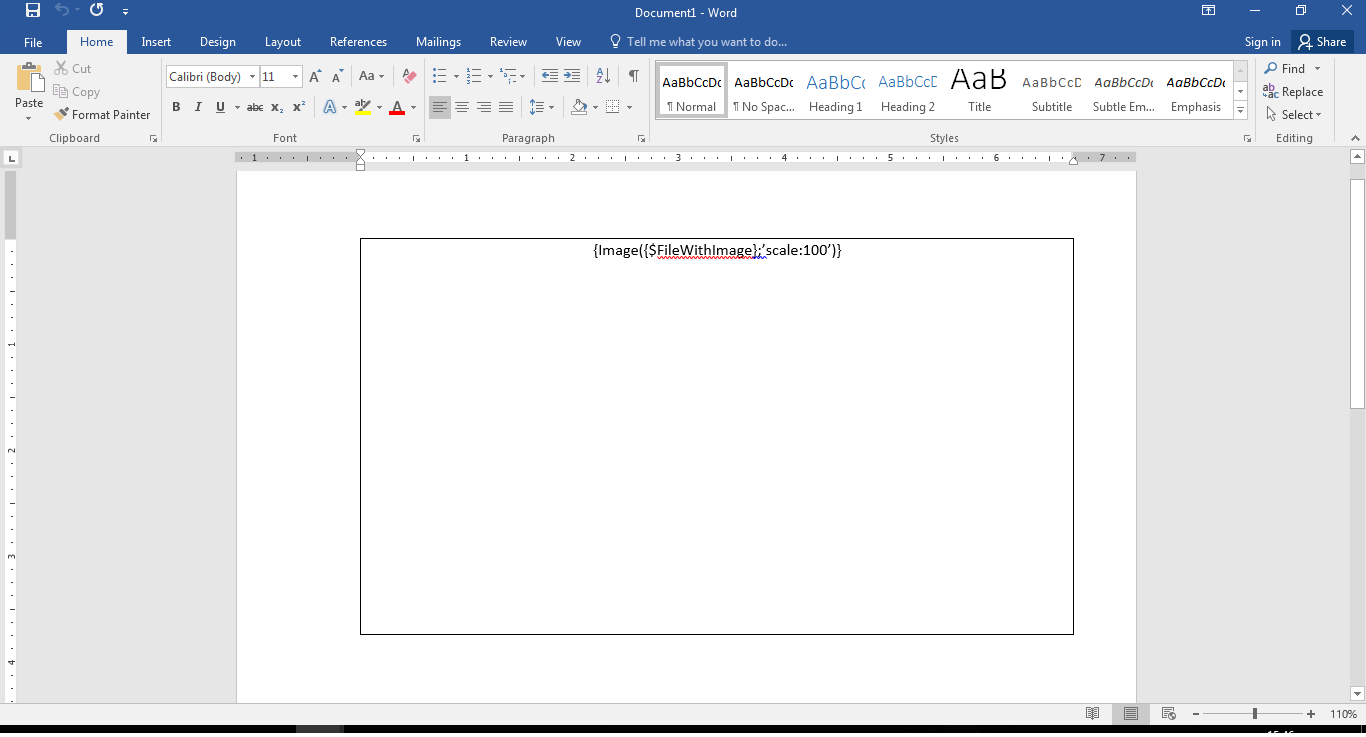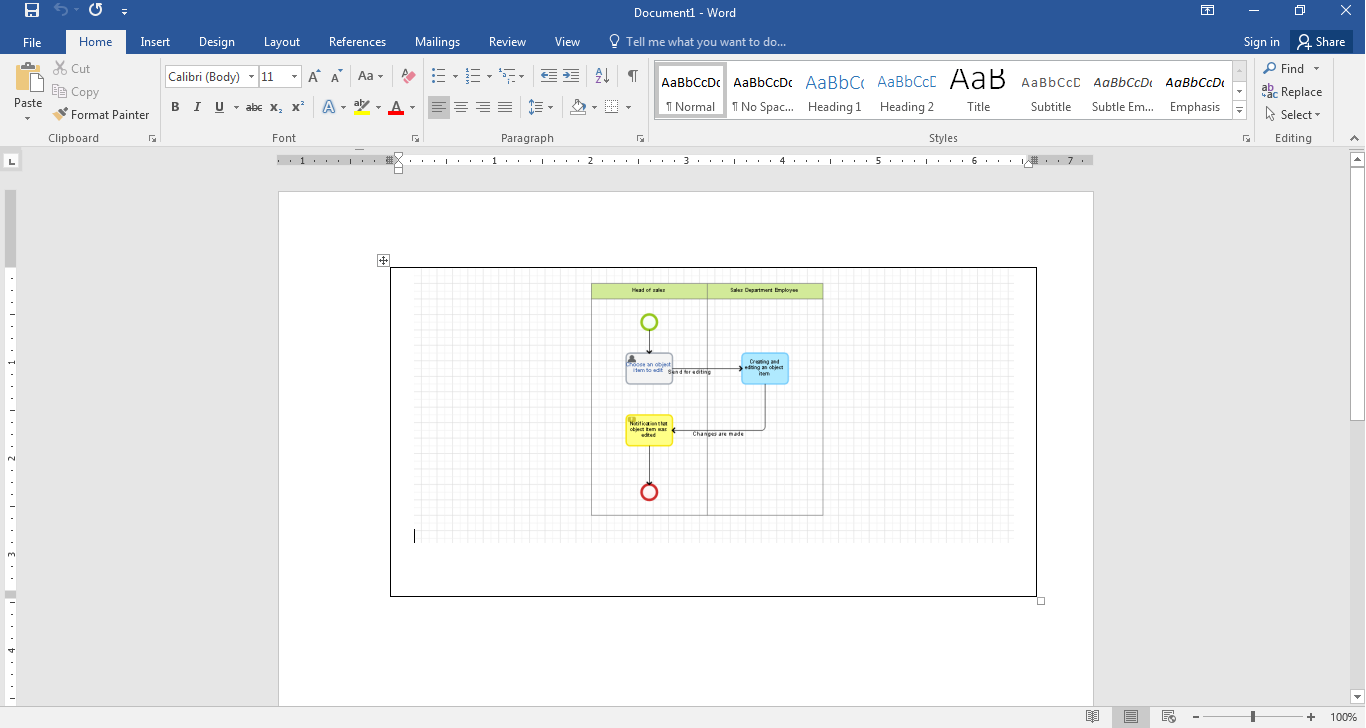# Script for making a fixed size image and inserting it into a document template

In this article you can find a script for generating an image that will be inserted in certain frames (in pixels). If the original image is beyond the fixed frames, it will be downsized without changing its aspect ratio. The largest size of an image is taken as the basis for the downsize scale calculation.

1. There is a template that contains a table. You need to insert an image that does not exceed the size of the table. Specify the context variable for the template in the table: {Image ({\$ FileWithImage}; ’scale: 100’)}2. Process graphic model looks as follows:In the first task, the original image is uploaded. After that, the script resizes the image according to the element of the table, then a document is generated from the template and in the last task you can see the result.

3. Script

Enable the global assemblies System.Drawing and Microsoft.CSharp in order to make the script work correctly.

Namespace:

``````using System;
using System.Collections.Generic;
using System.Linq;
using System.Text;
using EleWise.ELMA.API;
using EleWise.ELMA.Model.Common;
using EleWise.ELMA.Model.Entities;
using EleWise.ELMA.Model.Managers;
using EleWise.ELMA.Model.Types.Settings;
using EleWise.ELMA.Model.Entities.ProcessContext;
using System.Drawing;
using System.Drawing.Drawing2D;
using EleWise.ELMA.Runtime.Managers;
using EleWise.ELMA.Services;
using Microsoft.CSharp;
using EleWise.ELMA.Model.Services;
using EleWise.ELMA.Files;
``````

Script:

``````            //set the numbers – the size (in pixels) of the table into which you want to insert the image.
int tableWidth = 600;
int tableHeight = 600;
float nPercent = 1; //scaling factor
Image fromFile = Image.FromFile(context.Image.ContentFilePath);
var imageHeight = fromFile.Size.Height;
var imageWidth = fromFile.Size.Width;
// if the image does not fit the specified size, downsize it
if (imageHeight > tableHeight | imageWidth > tableWidth)
{
// determine the side of the image, which is scaled
if (imageHeight > imageWidth)
{
nPercent = (float)tableHeight / (float)imageHeight;
}
else
{
nPercent = (float)tableWidth / (float)imageWidth;
}
int newWidth = System.Convert.ToInt32(imageWidth * nPercent);
int newHeight = System.Convert.ToInt32(imageHeight * nPercent);
//resizing
Bitmap newImage = new Bitmap(fromFile, new Size(newWidth, newHeight));
string path = context.Image.ContentFilePath + ".tmp";
newImage.Save(path);
//save the downsized image into the file
context.FileWithImage = InterfaceActivator.Create<BinaryFile>();
context.FileWithImage.Name = "image.png";
context.FileWithImage.CreateDate = DateTime.Now;
context.FileWithImage.InitializeContentFilePath();
System.IO.File.Copy(@path, context.FileWithImage.ContentFilePath);
Locator.GetServiceNotNull<IFileManager>().SaveFile(context.FileWithImage);
}
else
{
context.FileWithImage = context.Image;
}
``````

4. Result

When the process is completed, the following document will be generated: